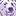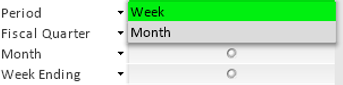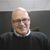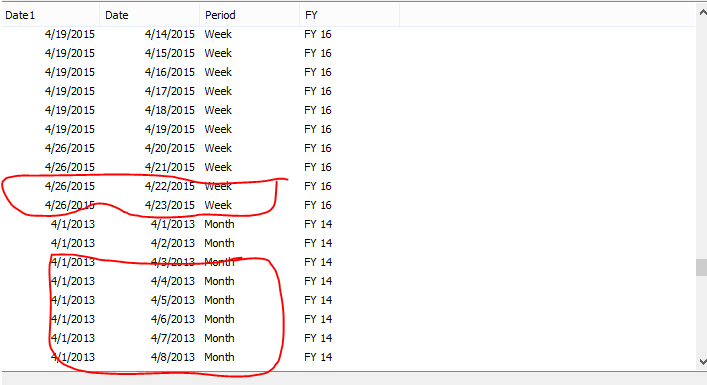# QlikView App Dev

Discussion Board for collaboration related to QlikView App Development.

cancel
Showing results for
Did you mean:Contributor II

## conversion of week and Month to Period

Hi i am a date qvd in which i am having

[Week End] and [Month]

week end has  only the weekends

Month Has only start of the month

i want to create a column as Period which has only two values in Month Or Week as in the below imageit has to be done in scripting level, where i had to use this in Multibox Resource Library

10 RepliesPartner - Champion III

You can create a table called Periods like this:

Periods:

LOAD * INLINE [

Period, %PeriodType

Week, 7

Month, 30

];

Then you can associate %PeriodType with other tables to use this as a filter or in a Set Expression.

The PeriodType is put in to have the option of linking as a key by a numeric key and to more efficiently

search by number instead of text in a Set Expression.Creator II

In the same load statement where you defined your date field add this:

if(monthstart(my_date) = date(my_date), 'Month', if(weekday(my_date) = 5 or weekday(my_date) = 6, 'Week')) as Period

Regards

MRContributor II
Author

Thank you
but i need a column like below one where ever the week date appears it should point as Week
if its month start it should point it has MOnth

in my data base i am having a data as below

for example

week end        period

4/5/2015           Week

11/5/2015          week

...

01/6/2015          MonthPartner - Champion III

How do you wish to determine how each row is considered week-end or month? The start of a month can be in a week-end so there is an overlap between the two. Then you must have some other logic that could tell whether this is a Week or a Month row... right?Partner - Champion III

Actually if you [Week End] field contains Nulls if it is not a Week-End and the [Month] contains Nulls if it is not a Month-row this logic should work:

....

If( Not(IsNull([week end])) , 'Week' , If( Not(IsNull([month])) , 'Month' ) ) AS period

....

If the source doesn't have Nulls but empty strings you would have to adjust it to this:

....

If( [week end]='' , 'Week' , [month]='' , 'Month' ) ) AS period

....

I would advise to use the helper table Periods as I suggested earlier and then the logic should be this:

....

If( Not(IsNull([week end])) , 7 , If( Not(IsNull([month])) , 30 ) ) AS %PeriodType

....Contributor II
Authorthe above image is my calender

where

i am having two variables

Let numWeek=num(curWeek);

Let numMonth=num(curMonth);

[GSS Week End] as Week, where [GSS Week End] < '\$(curWeek)';

[Month Start] as Month where [Month Start] < '\$(curMonth)';

This is the logic
but i dont know how make a column where period = week or monthLuminary Alumni

Hi,

Try like this

*

If(MonthStart(Weekend) = WeekEnd, 'Month', 'Week') AS Period

FROM DataSource;

Hope this helps you.

Regards,

jagan.Contributor II
Author

Hi its not working can you please suggest another one
i dont want to see the date column
where ever weekly data is there it should be named as week
and monthly data as Month
both week and month should be sitting in a column PeriodContributor II
Author

HI need to create a extra column in calender which has

week(TempDate) As week

Month(TempDate) As Month,

// one more column has Period (which should have only week or month)

as in below screen shot where ever month starts it should be month

if not it should week

Where Date 1 is Week end / week,